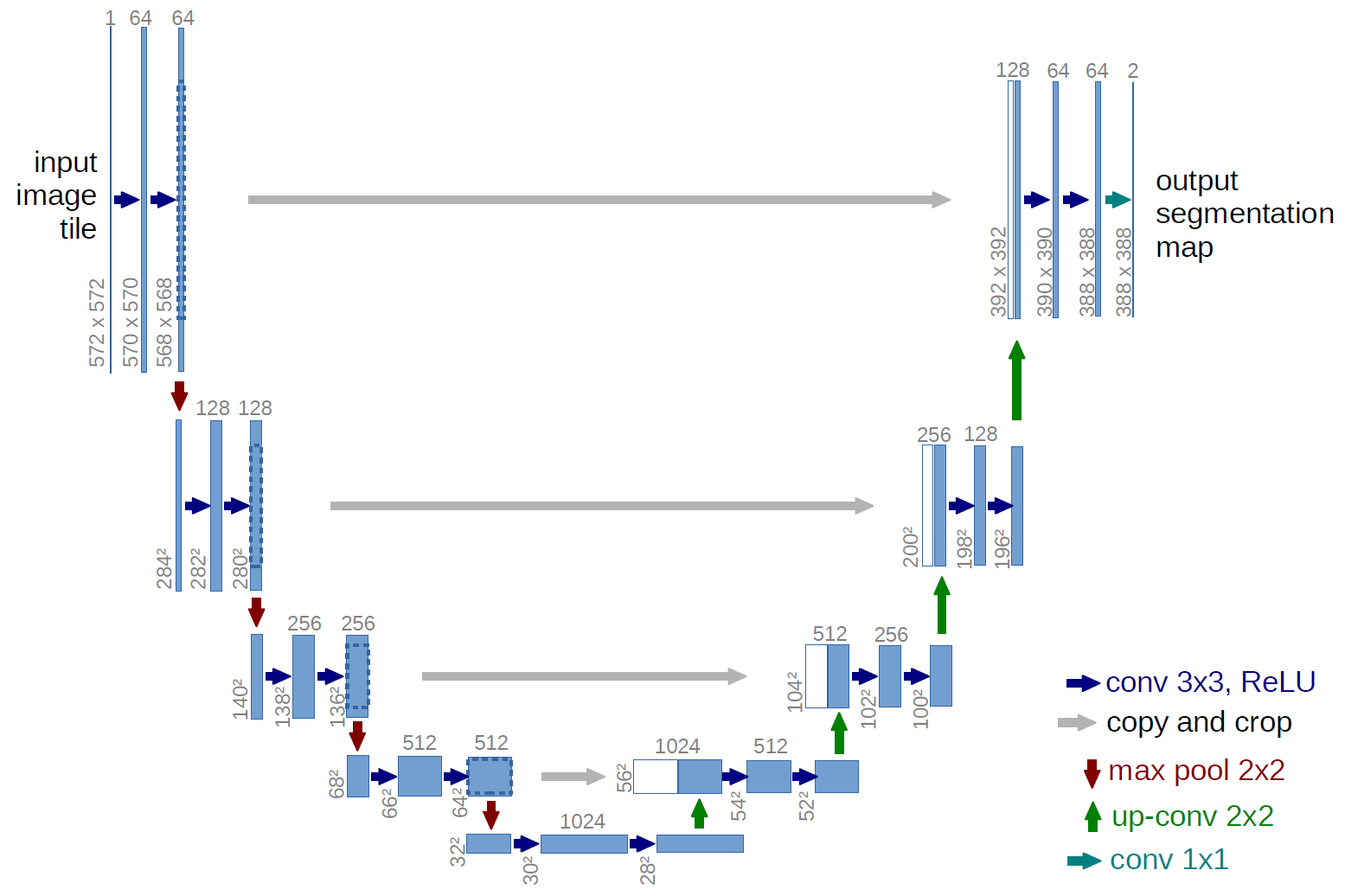# U-Net convolutional neural network

I am currently trying to understand how exactly the U-Net (https://lmb.informatik.uni-freiburg.de/people/ronneber/u-net/) works and so far failed to understand some key points, which are the following:

• What is the purpose of using two convolutional layers in a row?
• Why does the max pooling operation double the number of feature channels?
• What is the purpose of merging the feature maps from the contracting and expanding path?

Could somebody please elaborate these points above?

• Regarding your first question: multiple 3x3 convs were introduced in VGG networks. Main benefit is that two 3x3 convolutions have same reach as 5x5 convolution but with only 2*3*3 = 18 params instead of 25. – Tautvydas Sep 13 '18 at 14:06

As a brief refresher, U-Net refers to the following architecture by Ronneberger, Fischer and Brox (2015):What is the purpose of using two convolutional layers in a row?

Around the time U-Net paper was published, this was quite a common practice. See for example other papers following the same pattern [1,2]. I think there is actually a paper describing that putting two convolutional layers between pooling works better than using only one, but unfortunately I cannot remember which paper it is. Note that each of these convolution layers is followed by a non-linear activation, so they cannot be replaced by a single conv. layer.

Why does the max pooling operation double the number of feature channels?

I think you misinterpret the figure in the paper: A max-pooling layer does not change the number of the channels.

What is the purpose of merging the feature maps from the contracting and expanding path?

As you proceed to deeper layers, the features start representing "higher levels of abstraction", but with every pooling layer you are losing resolution. The layers at the end of the contracting path might be representing something like "There is such and such object in the image", but they do not contain information about the location of the object.

The expanding path effectively fuses the high-resolution details with the high-level semantic information. Note that similar approach was already used in the first fully convolutional networks .

: Springenberg, J.T., Dosovitskiy, A., Brox, T. and Riedmiller, M., 2014. Striving for simplicity: The all convolutional net. arXiv preprint arXiv:1412.6806.

: Simonyan, K. and Zisserman, A., 2014. Very deep convolutional networks for large-scale image recognition. arXiv preprint arXiv:1409.1556.

: Long, J., Shelhamer, E. and Darrell, T., 2015. Fully convolutional networks for semantic segmentation. In Proceedings of the IEEE conference on computer vision and pattern recognition (pp. 3431-3440).

• Thank you for this detailed answer to my questions. All is answered. – disputator1991 Sep 13 '18 at 14:43

One reason for using multiple filters together is that it reduces the number of parameters. This is because the parameters required for a 3x3 filter is 9 times the number of channels. The number of parameters needed for a 7x7 filter is 49 times the number of channels. 3 x 9 < 49, so using 3 3x3 channels uses fewer parameters than 1 7x7 channel. Source.